Next: Exercises Up: Newton's Law of Cooling Previous: Newton's Law of Cooling

### Example

A cup of coffee is poured from a potwhose contents areinto a non-insulated cup in a room at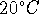. After a minute, the coffee has cooled to. How much time is required before the coffee reaches a drinkable temperature of?

1. Enter the equation.
```  > eq1:= T = exp(k*t+C) +20;
```2. Solve for C by using the initial temperature.
```  > eq2:= subs({t=0,T=95},eq1);
``````  > C:=evalf(solve(eq2,C));
```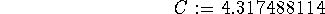3. Solve for k by using the temperature at t=1.
```  > eq3:=subs({t=1,T=90},eq1);
```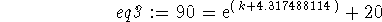```  > k:=solve(eq3,k);
```4. Look at the original equation and notice that the only variable is t. Proceed to enter desired temperature and solve for t.
```  > eq1;
```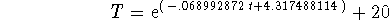```  > eq4:=subs(T=65,eq1);
```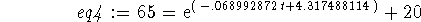```  > solve(eq4,t);
```The time it takes for the coffee to reachis 7.4 minutes.

Roxanne Tisch
Tue Sep 24 13:59:38 EDT 1996